# First Nine Weeks Review JENKINS Place Value Reasons

• Slides: 15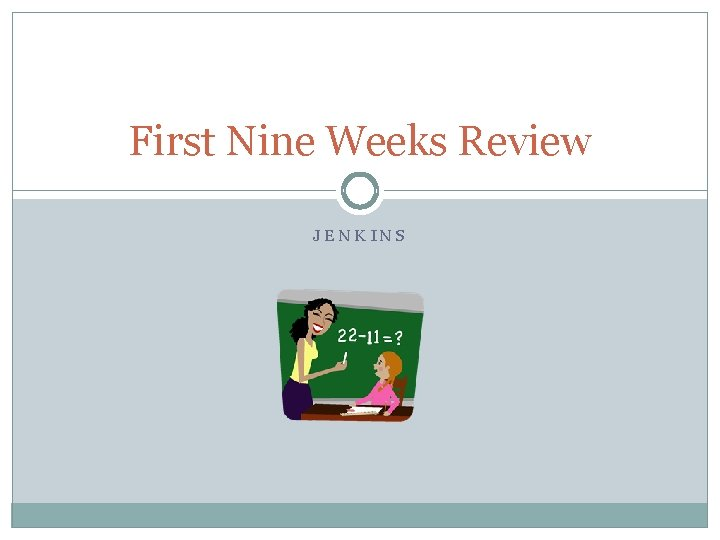First Nine Weeks Review JENKINSPlace Value Reasons why place value is so important… Place value helps us to line up numbers properly so that we are able to add and subtract correctly. Place value helps us to understand if a number is greater than or less than Place value helps us to understand base 10 and the whole on numeration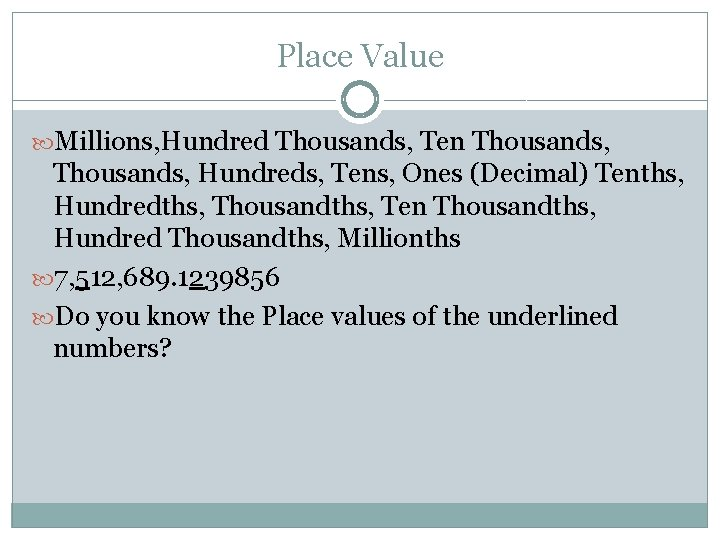Place Value Millions, Hundred Thousands, Ten Thousands, Hundreds, Tens, Ones (Decimal) Tenths, Hundredths, Thousandths, Ten Thousandths, Hundred Thousandths, Millionths 7, 512, 689. 1239856 Do you know the Place values of the underlined numbers?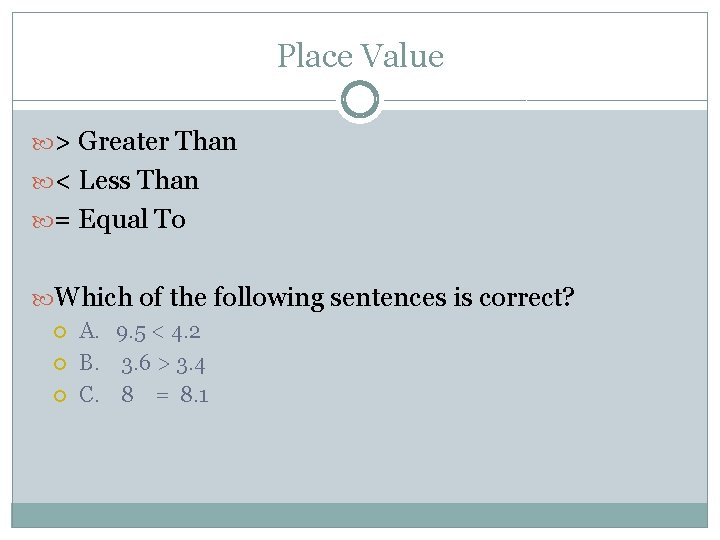Place Value > Greater Than < Less Than = Equal To Which of the following sentences is correct? A. 9. 5 < 4. 2 B. 3. 6 > 3. 4 C. 8 = 8. 1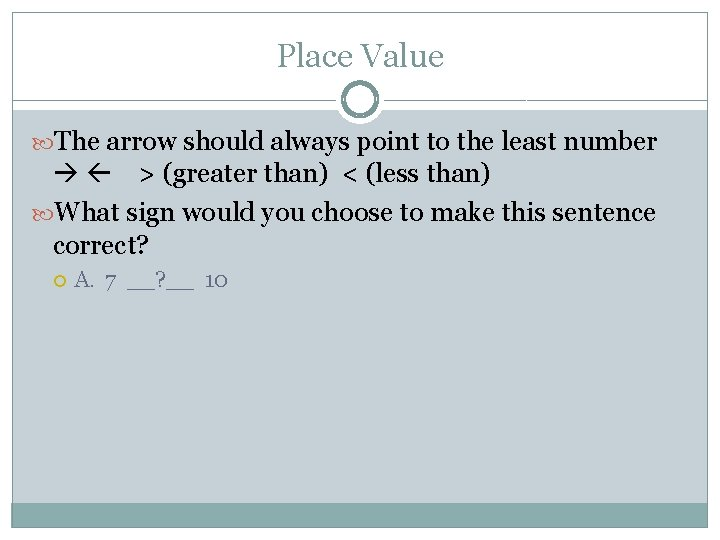Place Value The arrow should always point to the least number > (greater than) < (less than) What sign would you choose to make this sentence correct? A. 7 __? __ 10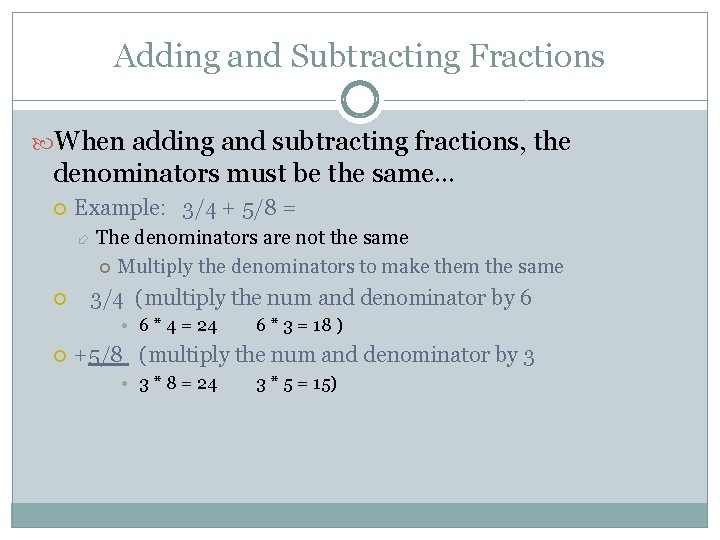Adding and Subtracting Fractions When adding and subtracting fractions, the denominators must be the same… Example: 3/4 + 5/8 = The denominators are not the same Multiply the denominators to make them the same 3/4 (multiply the num and denominator by 6 • 6 * 4 = 24 6 * 3 = 18 ) +5/8 (multiply the num and denominator by 3 • 3 * 8 = 24 3 * 5 = 15)Adding and Subtracting Fractions Cont. 3/4 = 18/24 + 5/8 = 15/24 33/24 = 11/8 (lowest terms)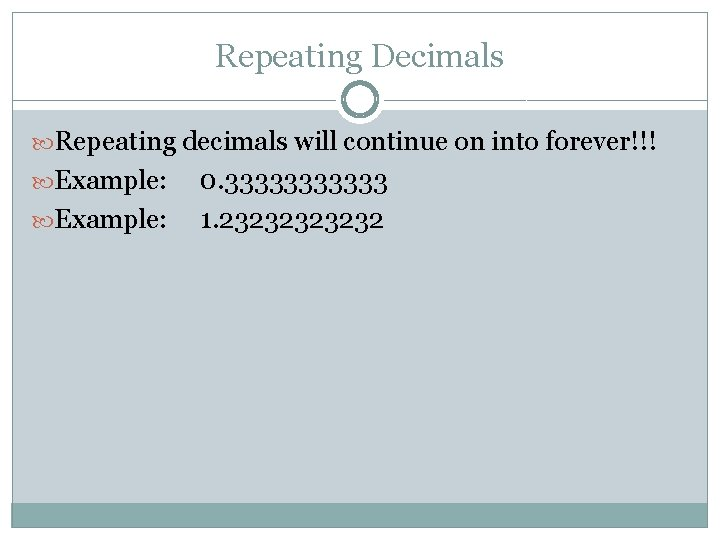Repeating Decimals Repeating decimals will continue on into forever!!! Example: 0. 333333 1. 2323232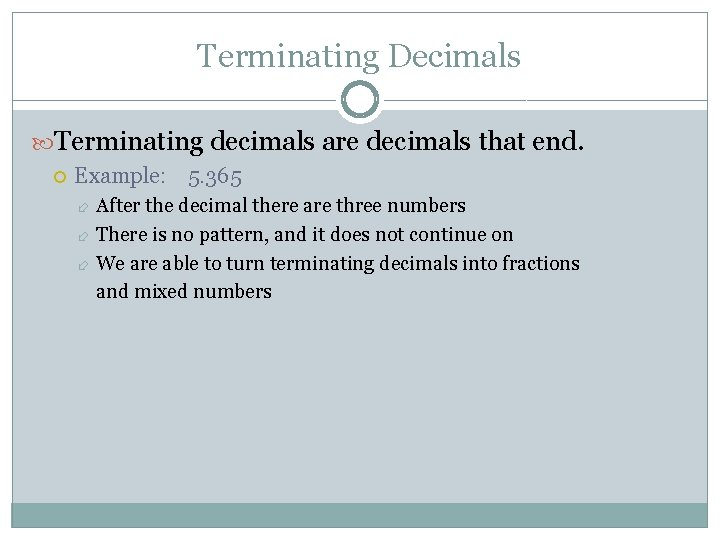Terminating Decimals Terminating decimals are decimals that end. Example: 5. 365 After the decimal there are three numbers There is no pattern, and it does not continue on We are able to turn terminating decimals into fractions and mixed numbers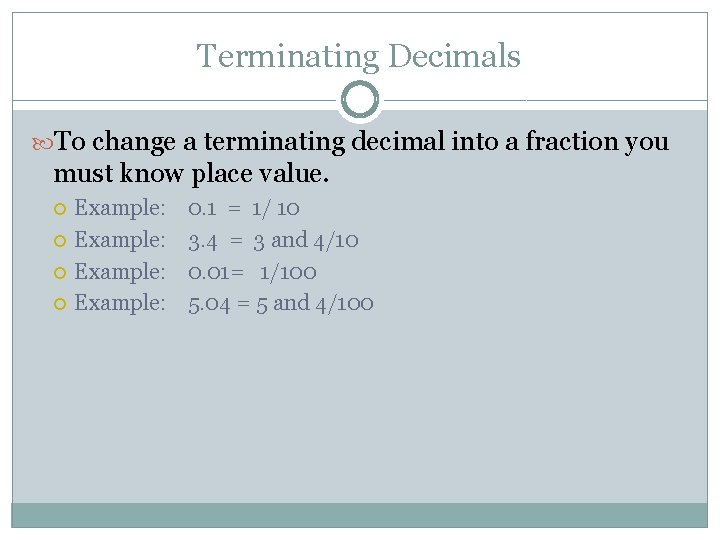Terminating Decimals To change a terminating decimal into a fraction you must know place value. Example: 0. 1 = 1/ 10 3. 4 = 3 and 4/10 0. 01= 1/100 5. 04 = 5 and 4/100Converting Fractions into Decimals Divide the fraction The bottom number (denominator) will be your outside number The top number (numerator) will be the inside number Example: ¾ = 4 divided by 3 =. 75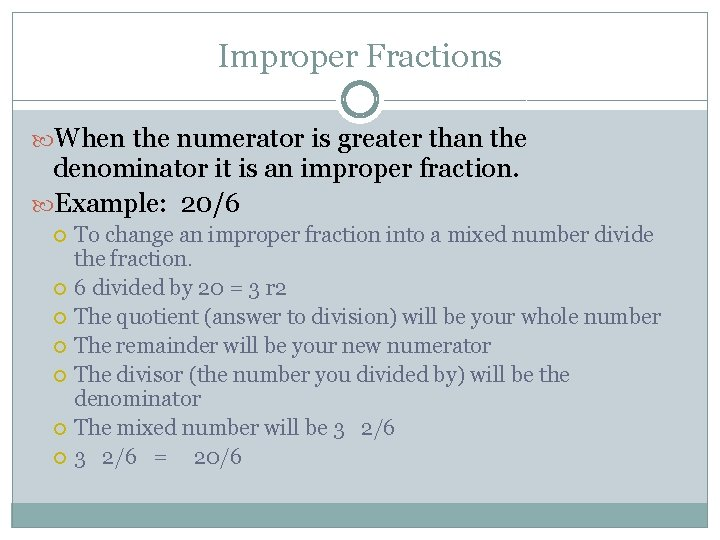Improper Fractions When the numerator is greater than the denominator it is an improper fraction. Example: 20/6 To change an improper fraction into a mixed number divide the fraction. 6 divided by 20 = 3 r 2 The quotient (answer to division) will be your whole number The remainder will be your new numerator The divisor (the number you divided by) will be the denominator The mixed number will be 3 2/6 = 20/6Mixed Number A mixed number is a whole number and a fraction. Example: 3 ½ Convert this mixed number into an improper fraction by multiplying the denominator and the whole number then add the numerator 2 * 3 = 6 + 1 = 7 7 is the new numerator The denominator stays the same 7/2 = 3 ½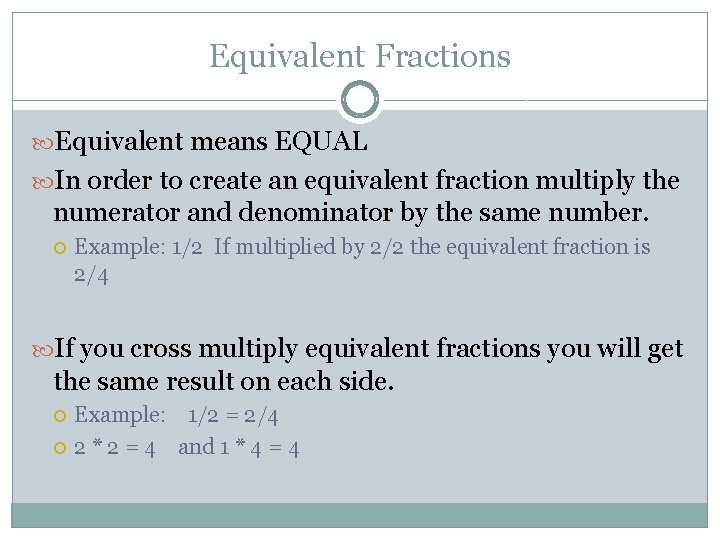Equivalent Fractions Equivalent means EQUAL In order to create an equivalent fraction multiply the numerator and denominator by the same number. Example: 1/2 If multiplied by 2/2 the equivalent fraction is 2/4 If you cross multiply equivalent fractions you will get the same result on each side. Example: 1/2 = 2/4 2 * 2 = 4 and 1 * 4 = 4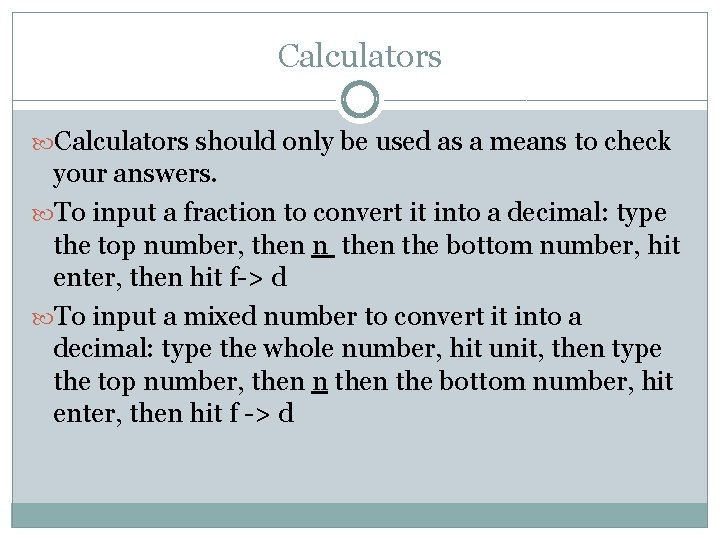Calculators should only be used as a means to check your answers. To input a fraction to convert it into a decimal: type the top number, then n the bottom number, hit enter, then hit f-> d To input a mixed number to convert it into a decimal: type the whole number, hit unit, then type the top number, then n the bottom number, hit enter, then hit f -> d